# Non Verbal Reasoning - Classification

### Exercise :: Classification - Section 2

In each problem, out of the five figures marked (1), (2), (3), (4) and (5), four are similar in a certain manner. However, one figure is not like the other four. Choose the figure which is different from the rest.

16.

Choose the figure which is different from the rest.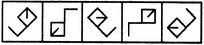(1)     (2)     (3)     (4)     (5)

 A. 1 B. 2 C. 3 D. 4 E. 5

Explanation:

The line segment inside the square is attached to the corner which lies opposite to the corner to which the outer L-shaped element is attached.

17.

Choose the figure which is different from the rest.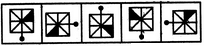(1)     (2)     (3)     (4)     (5)

 A. 1 B. 2 C. 3 D. 4 E. 5

Explanation:

All other figures can be rotated into each other.

18.

Choose the figure which is different from the rest.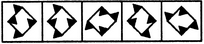(1)     (2)     (3)     (4)     (5)

 A. 1 B. 2 C. 3 D. 4 E. 5

Explanation:

Only in fig. (4), each side of the square has at one black triangle attached to it.

19.

Choose the figure which is different from the rest.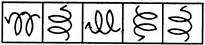(1)     (2)     (3)     (4)     (5)

 A. 1 B. 2 C. 3 D. 4 E. 5

Explanation:

All other figures can be rotated into each other.

20.

Choose the figure which is different from the rest.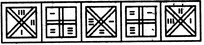(1)     (2)     (3)     (4)     (5)

 A. 1 B. 2 C. 3 D. 4 E. 5